Basics of Geometry
edHelper subscribers - Create a new printable

Number of Keys
 Select the number of different printables: 1 key 2 keys 3 keys 4 keys 5 keys

Number of Pages (for each key)
 Select the number of pages: 1 page 2 pages 3 pages 4 pages

Include an answer key (answer keys will be at the end of the printable)
High School Geometry
High School Geometry
Math

 Name _____________________________Date ___________________ (Key 1 - Answer ID # 0183892)
1.

In the diagram,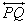and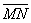intersect at the midpoint of. Which of the following statements are NOT true?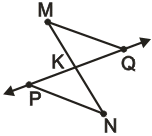(I)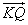and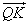are opposite rays.
(II)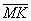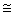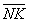(III) MQ = PN
(IV)is the same as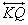.
(V) QK + KM = MQ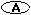I and V only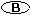I, III, and V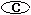III and IV only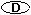III, IV, and V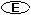II and III only

2.

Devin and Sierra were playing a learning game in which they had to virtually sort points, lines, and planes from a collection of points that appeared on an electronic game board. For a correct response, the board flashed green. If an incorrect response was given, or if a duplicate of a previous response was offered, the board would buzz and flash red. Pretend you are playing the game and using the points below. Name 3 points, 3 lines, and 3 planes. Note: The points do not all necessarily lie in the same plane.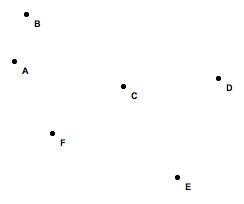3.

Suppose M is between L and N. LM = (1/2y + 3), MN = (4y + 7/2), LN = 135. Find the value of y.

4.

Two angles are complements. 30less than twice the larger angle equals twice the smaller angle. Find the measures of the two angles.

Basics of Geometry
edHelper subscribers - Create a new printable

Number of Keys
 Select the number of different printables: 1 key 2 keys 3 keys 4 keys 5 keys

Number of Pages (for each key)
 Select the number of pages: 1 page 2 pages 3 pages 4 pages